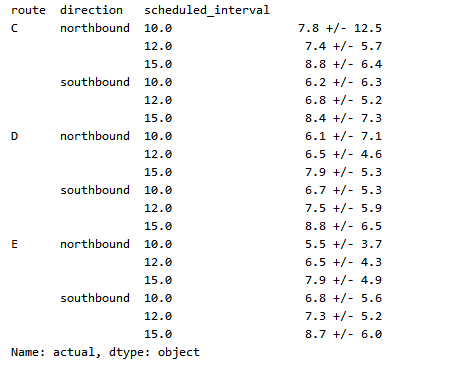>科技>正文

# 公交车总迟到？你大概掉进了等待时间悖论importnumpyasnpN =1000000# number of busestau =10# average minutes between arrivalsrand = np.random.RandomState(42) # universal random seedbus_arrival_times = N * tau * np.sort(rand.rand(N))

intervals = np.diff(bus_arrival_times)intervals.mean()

9.9999879601518398

defsimulate_wait_times(arrival_times, rseed=8675309, # Jenny's random seed n_passengers=1000000): rand = np.random.RandomState(rseed) arrival_times = np.asarray(arrival_times) passenger_times = arrival_times.max() * rand.rand(n_passengers) # find the index of the next bus for each simulated passenger i = np.searchsorted(arrival_times, passenger_times, side='right') return arrival_times[i] - passenger_times

wait_times = simulate_wait_times(bus_arrival_times)wait_times.mean()

10.001584206227317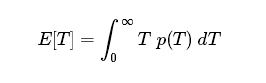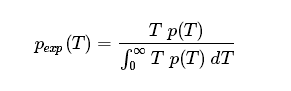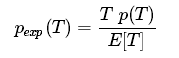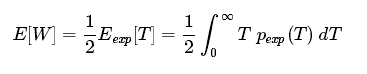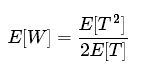%matplotlib inlineimportmatplotlib.pyplotaspltplt.style.use('seaborn')plt.hist(intervals, bins=np.arange(80), density=True)plt.axvline(intervals.mean(), color='black', linestyle='dotted')plt.xlabel('Interval between arrivals (minutes)')plt.ylabel('Probability density');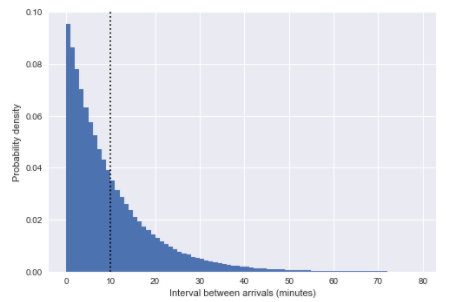fromscipy.statsimportpoisson# count the number of arrivals in 1-hour binsbinsize =60binned_arrivals = np.bincount((bus_arrival_times // binsize).astype(int))x = np.arange(20)# plot the resultsplt.hist(binned_arrivals, bins=x -0.5, density=True, alpha=0.5, label='simulation')plt.plot(x, poisson(binsize / tau).pmf(x),'ok', label='Poisson prediction')plt.xlabel('Number of arrivals per hour')plt.ylabel('frequency')plt.legend();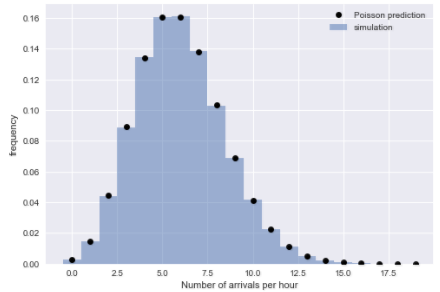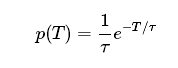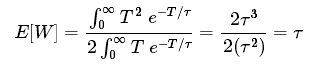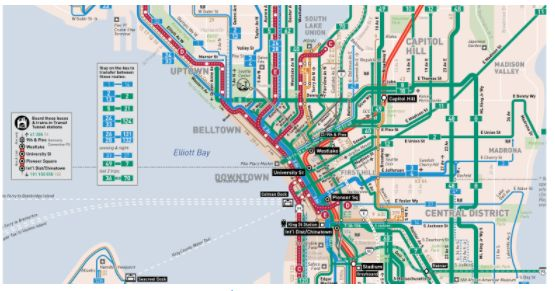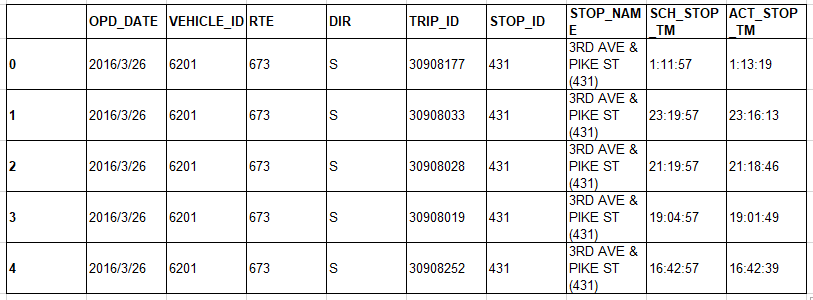# combine date and time into a single timestampdf['scheduled'] = pd.to_datetime(df['OPD_DATE'] +' '+ df['SCH_STOP_TM'])df['actual'] = pd.to_datetime(df['OPD_DATE'] +' '+ df['ACT_STOP_TM'])# if scheduled & actual span midnight, then the actual day needs to be adjustedminute = np.timedelta64(1,'m')hour =60* minutediff_hrs = (df['actual'] - df['scheduled']) / hourdf.loc[diff_hrs >20,'actual'] -=24* hourdf.loc[diff_hrs <-20,'actual'] +=24* hourdf['minutes_late'] = (df['actual'] - df['scheduled']) / minute# map internal route codes to external route lettersdf['route'] = df['RTE'].replace({673:'C',674:'D',675:'E'}).astype('category')df['direction'] = df['DIR'].replace({'N':'northbound','S':'southbound'}).astype('category')# extract useful columnsdf = df[['route','direction','scheduled','actual','minutes_late']].copy()df.head()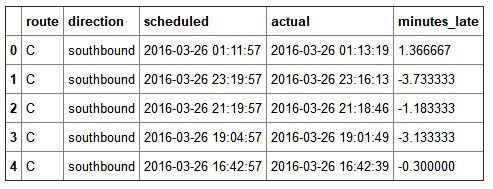importseabornassnsg = sns.FacetGrid(df, row="direction", col="route")g.map(plt.hist,"minutes_late", bins=np.arange(-10,20))g.set_titles('{col_name} {row_name}')g.set_axis_labels('minutes late','number of buses');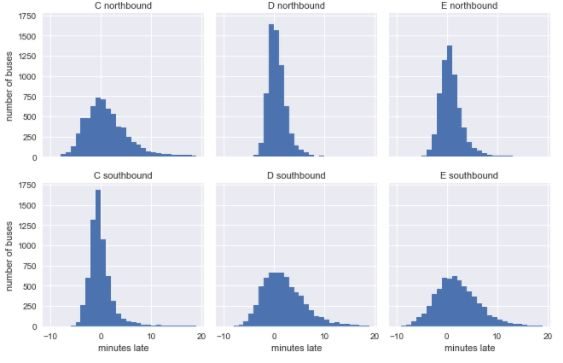defcompute_headway(scheduled): minute = np.timedelta64(1,'m')returnscheduled.sort_values().diff() / minutegrouped = df.groupby(['route','direction'])df['actual_interval'] = grouped['actual'].transform(compute_headway)df['scheduled_interval'] = grouped['scheduled'].transform(compute_headway)g = sns.FacetGrid(df.dropna(), row="direction", col="route")g.map(plt.hist,"actual_interval", bins=np.arange(50) +0.5)g.set_titles('{col_name} {row_name}')g.set_axis_labels('actual interval (minutes)','number of buses');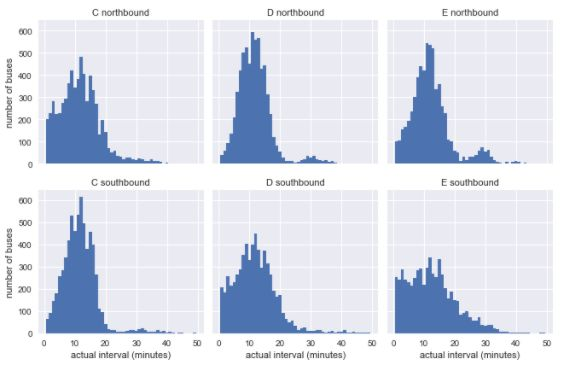g = sns.FacetGrid(df.dropna(), row="direction", col="route")g.map(plt.hist,"scheduled_interval", bins=np.arange(20) -0.5)g.set_titles('{col_name} {row_name}')g.set_axis_labels('scheduled interval (minutes)','frequency');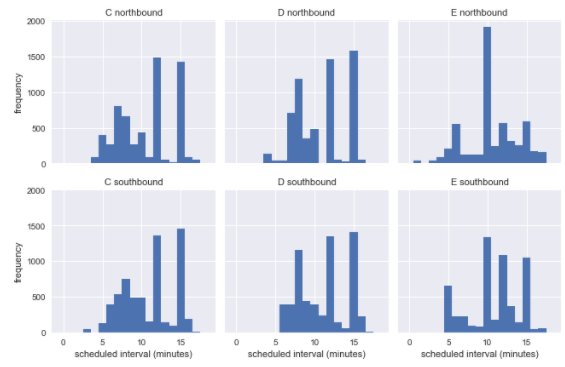defstack_sequence(data):# first, sort by scheduled time data = data.sort_values('scheduled')# re-stack data & recompute relevant quantities data['scheduled'] = data['scheduled_interval'].cumsum() data['actual'] = data['scheduled'] + data['minutes_late'] data['actual_interval'] = data['actual'].sort_values().diff()returndatasubset = df[df.scheduled_interval.isin([10,12,15])]grouped = subset.groupby(['route','direction','scheduled_interval'])sequenced = grouped.apply(stack_sequence).reset_index(drop=True)sequenced.head()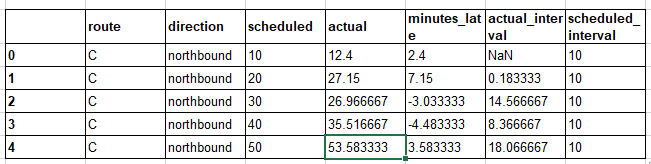forroutein['C','D','E']: g = sns.FacetGrid(sequenced.query(f"route == '{route}'"), row="direction", col="scheduled_interval") g.map(plt.hist,"actual_interval", bins=np.arange(40) +0.5) g.set_titles('{row_name} ({col_name:.0f} min)') g.set_axis_labels('actual interval (min)','count') g.fig.set_size_inches(8,4) g.fig.suptitle(f'{route} line', y=1.05, fontsize=14)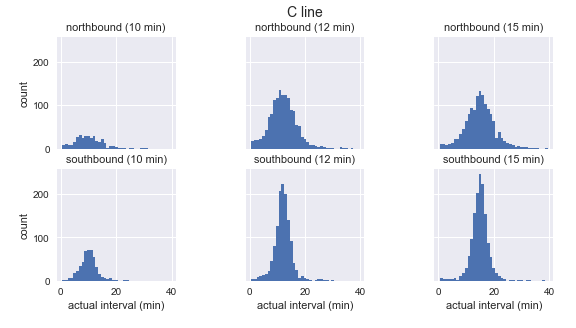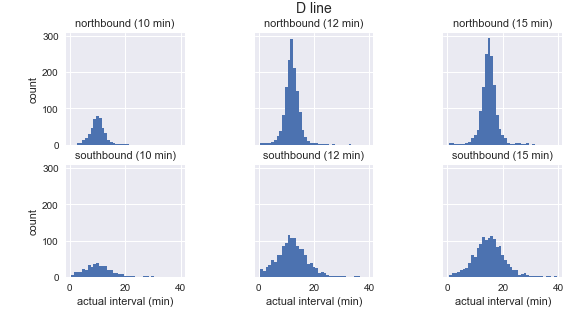grouped = sequenced.groupby(['route','direction','scheduled_interval'])sims = grouped['actual'].apply(simulate_wait_times)sims.apply(lambdatimes:"{0:.1f} +/- {1:.1f}".format(times.mean(), times.std()))